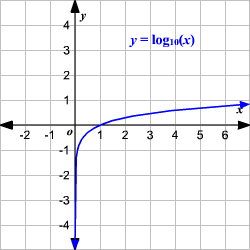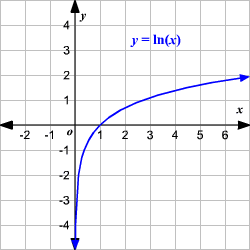# Logarithmic Functions

The "basic" logarithmic function is the function, $y={\mathrm{log}}_{b}x$, where $x$, $b>0$ and $b\ne 1$.

The graph of the logarithmic function $y=\mathrm{log}x$ is shown. (Remember that when no base is shown, the base is understood to be $10$.)Observe that the logarithmic function $f\left(x\right)={\mathrm{log}}_{b}x$ is the inverse of the exponential function $g\left(x\right)={b}^{x}$. It has the following properties.

The domain is the set of all positive real numbers.

1. $f\left(x\right)={\mathrm{log}}_{b}x$ is not defined for negative values of $x$ , or for $0$ .

2. The range is the set of all real numbers.
(Since the logarithmic function is the inverse of the exponential function, the domain of logarithmic function is the range of exponential function, and vice versa.)

3. The function is continuous and one-to-one.

4. The $y$-axis is the asymptote of the graph.

5. The graph intersects the $x$-axis at $\left(1,0\right)$. That is, the $x$-intercept is $1$.

The logarithmic function, $y={\mathrm{log}}_{b}x$, can be shifted $k$ units vertically and $h$ units horizontally with the equation $y={\mathrm{log}}_{b}\left(x+h\right)+k$.

Vertical shift

If $k>0$, the graph would be shifted $k$ units up.

If $k<0$, the graph would be shifted $k$ units down.

Horizontal Shift

If $h>0$, the graph would be shifted $h$ units left.

If $h<0$, the graph would be shifted $h$ units right.

### Natural Logarithmic Function

The logarithm with base $e$ is called the natural logarithm. It is denoted by $\mathrm{ln}x$. The natural logarithmic function, $y=\mathrm{ln}x$, is the inverse of the natural base exponential function, $y={e}^{x}$.

The graph of the natural logarithmic function $y=\mathrm{ln}x$ is shown.Example:

Graph the function $y={\mathrm{log}}_{10}\left(x-1\right)+2$.

Start with the basic logarithmic graph $y={\mathrm{log}}_{10}x$ or $y=\mathrm{log}x$. Then shift the graph $1$ unit to the right and $2$ units up.